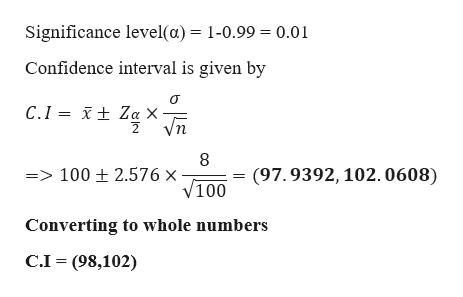To determine how well a new method of teaching vocabulary is working in a certain elementary school, education researchers plan to give a vocabulary test to a sample of 100 sixth graders. It is known that scores on this test have a standard deviation of 8. The researchers found the sample mean to be 100. Construct a 99% confidence interval for the population mean. Round your answers to whole numbers.

Question
To determine how well a new method of teaching vocabulary is working in a certain elementary school, education researchers plan to give a vocabulary test to a sample of 100 sixth graders. It is known that scores on this test have a standard deviation of 8. The researchers found the sample mean to be 100. Construct a 99% confidence interval for the population mean. Round your answers to whole numbers.
Step 1

Given data

Sample size(n) = 100

Sample mean = 100

Population standard deviat...help_outlineImage TranscriptioncloseSignificance level(a) = 1-0.99 = 0.01 Confidence interval is given by t Za X Vn C.I 8 => 100 ± 2.576 x V100 (97.9392, 102.0608) Converting to whole numbers C.I (98,102) fullscreen

Want to see the full answer?

See Solution

Want to see this answer and more?

Our solutions are written by experts, many with advanced degrees, and available 24/7

See Solution
Tagged in

Hypothesis Testing